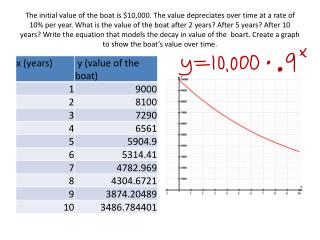DownloadDownload PresentationQuestion 2

# Question 2

Télécharger la présentation## Question 2

- - - - - - - - - - - - - - - - - - - - - - - - - - - E N D - - - - - - - - - - - - - - - - - - - - - - - - - - -
##### Presentation Transcript

1. The initial value of the boat is \$10,000. The value depreciates over time at a rate of 10% per year. What is the value of the boat after 2 years? After 5 years? After 10 years? Write the equation that models the decay in value of the boart. Create a graph to show the boat’s value over time.

2. Question 2 China’s one-child policy was implemented with a goal of reducing China’s population to 700 million by 2050. China’s 2000 population is about 1.2 billion. Suppose that China’s population declines at a rate of 0.5% per year. What will China’s population be in 2010, 2020, 2030, 2040, and 2050? (Note: use the year 2000 as x=0, then count years from there).

3. Question 3 Suppose you deposit \$100 into an account that earns 5% annual interest. Interest is paid once at the end of year. You do not make additional deposits or withdrawals. Make a graph showing how much money you will have over the next 20 years. When will your investment be double the original amount?

4. Finding the exponential equation • Determine the initial value (the value of y when x=0). This is a. • Look at the y values for x=0 and x=1 y1/y0=b